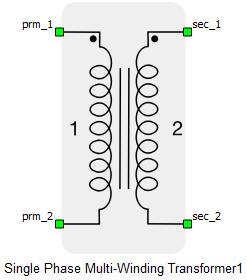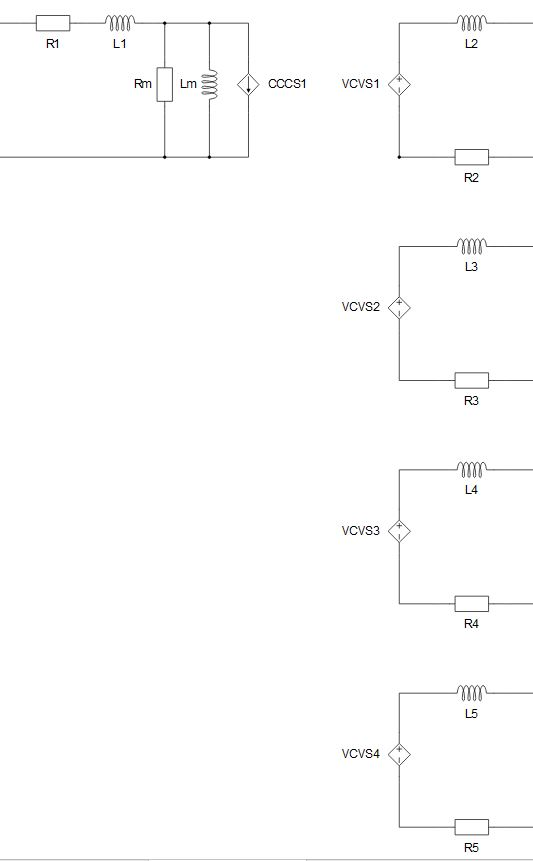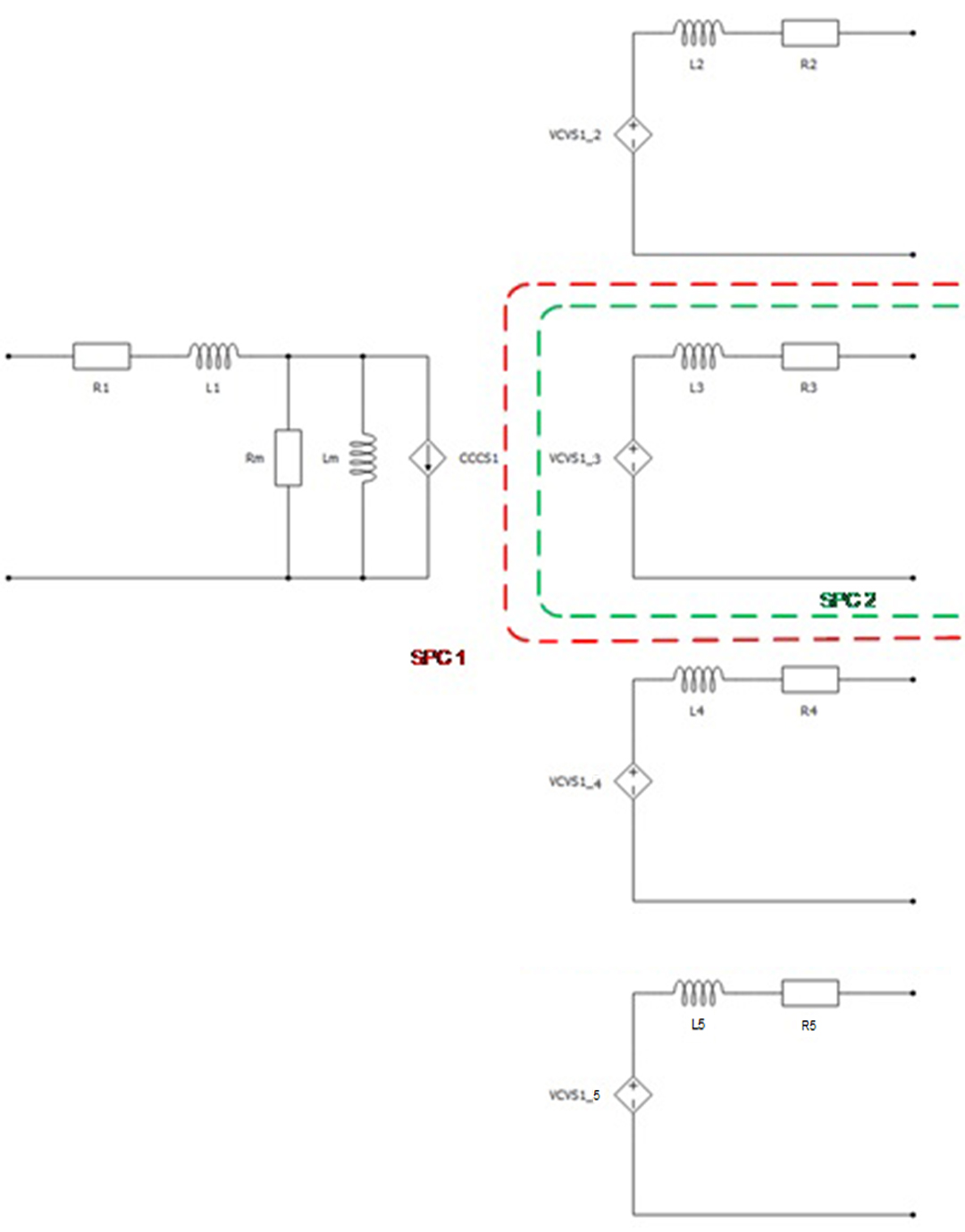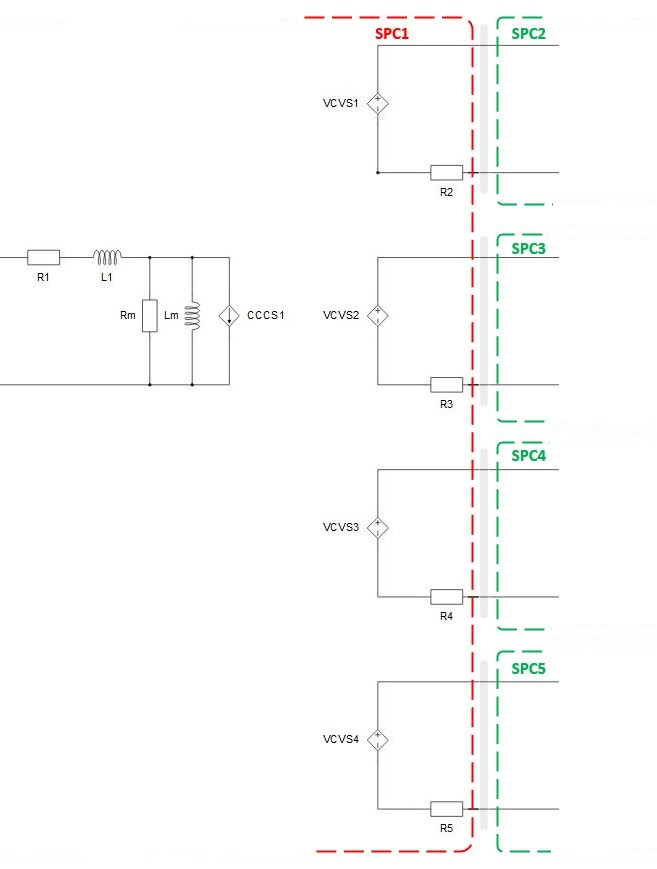# Single phase multi-winding transformer

This section describes single phase multi-winding transformer

The single phase multi-winding transformer component models two to ten coupled windings on the same core. The magnetization inductance Lm can be linear or with saturation, it is modeled on the primary side of the transformer. Core losses are modeled as Rm resistance located on the primary side of the transformer. Also, it is possible to neglect Lm and Rm by selecting Lm/Rm neglected in the Core model property. For more informations, please refer to Core model

The single phase multi-winding transformer schematic symbol and input parameters are given in Table 1.

Table 1. The single phase multi-winding transformer component in the HIL Toolbox.
component component dialog window component parameters• Input parameters option – SI/p.u.
• Number of windings (2 to 10)
• Nominal Power of the transformer in VA (Sn)
• Nominal frequency in Hz (fn)
• Number of turns of first (primary) winding (n prim)
• Number of turns of secondary winding(s) (n sec)
• Leakage inductance of first(primary) winding (L prim)
• Initial current in primary winding (I prim)
• Leakage inductance of secondary winding(s) (L sec)
• Initial current in secondary winding(s) (I sec)
• Resistance of primary winding(R prim)
• Resistance of secondary winding(s) (R sec)
• Core model - Linear, Non-Linear, Rm/Lm neglected
• Magnetization Inductance (Lm)
• Equivalent resistance representing the iron core losses (Rm)
• Import from PU button• Embedded coupling 1-2 - inserts a coupling component (Ideal Transformer based or TLM) between winding 1 and 2.
• Embedded coupling 1-3 - inserts a coupling component (Ideal Transformer based or TLM) between winding 1 and 3.
• Embedded coupling 1-4 - inserts a coupling component (Ideal Transformer based or TLM) between winding 1 and 4.
• Embedded coupling 1-5 - inserts a coupling component (Ideal Transformer based or TLM) between winding 1 and 5.
• TLM 1-2/Embedded components ratio - Defines how coupling to embedded inductors ratio will be calculated. Related to coupling between winding 1 and 2. Available only for the TLM coupling.
• TLM 1-3/Embedded components ratio - Defines how coupling to embedded inductors ratio will be calculated. Related to coupling between winding 1 and 3. Available only for the TLM coupling.
• TLM 1-4/Embedded components ratio - Defines how coupling to embedded inductors ratio will be calculated. Related to coupling between winding 1 and 4. Available only for the TLM coupling.
• TLM 1-5/Embedded components ratio - Defines how coupling to embedded inductors ratio will be calculated. Related to coupling between winding 1 and 5. Available only for the TLM coupling.
• Ratio - Used to specify coupling to embedded inductors ratio if Manual option is selected in TLM/Embedded components ratio property.

Number of windings - The user can choose the number of windings (2 - 10).

Parameters for secondary side properties (V sec, R sec, L sec, I sec) must be set as a vector with the length of either one or the number of secondary windings.

A schematic block diagram of the single phase multi-winding transformer (number of windings is set to five) block with the corresponding component arrangement and naming is shown in The schematic block diagram of a single phase multi-winding transformer with corresponding component naming.Figure 1. The schematic block diagram of a single phase multi-winding transformer with corresponding component naming

## Embedded coupling

There are two possible options for embedded coupling in the single phase four winding transformer, Ideal Transformer based coupling and TLM coupling.

If Embedded coupling is set to Ideal Transformer, Ideal Transformer based coupling will be placed between two windings of transformer.

In Figure 2 and Figure 3, respectively, are given visual representations of division of circuit among standard processing cores, in case where Embedded coupling 1-3 is set to Ideal Transformer and in case where e.g. five embedded couplings are set to Ideal Transformer.Figure 2. Representation of circuit division between cores where Embedded coupling 1-3 is set to 'Ideal Transformer'Figure 3. Representation of circuit division among cores where all five couplings are set to 'Ideal Transformer'

If Embedded coupling is set to TLM, secondary winding inductor will be replaced with TLM coupling component. Inductance will be divided between coupling and embedded inductors (inductors will be hidden in the TLM). TLM to embedded inductors ratio can be determined by compiler, but also it can be specified explicitly. If Automatic option is selected, ratio will be determined by discretization method. If Manual option is selected, ratio can be explicitly set to meet user requirements. For more information on TLM couplings please refer to Core couplings - TLM.

In Figure 4 and Figure 5, respectively, are given visual representations of division of circuit among standard processing cores, in case where Embedded coupling 1-3 is set to TLM and in case where eg. five embedded couplings are set to TLM.Figure 4. Representation of circuit division between cores where Embedded coupling 1-3 is set to 'TLM'Figure 5. Representation of circuit division among cores where all five Embedded couplings are set to 'TLM'

## State variable naming for the single phase multi-winding transformer (internal to the component)

Analog output variable name Description
(name).L prim Primary winding current
(name).L sec Secondary winding(s) current
(name).Lm Magnetizing current